Xiao Lu1, Xinzheng Lu1, [*] , Hong Guan2 and Lieping Ye1 1 Key Laboratory of Civil Engineering Safety and Durability of China Education Ministry, Department of Civil Engineering, Tsinghua University, Beijing, P.R. China, 100084;  2Griffith School of Engineering, Griffith University Gold Coast Campus, Queensland 4222, Australia Advances in Structural Engineering, 2013, 16(7): 1249-1262. Download Full Text Abstract: Ground motion intensity measures (IMs) are an important basis of structural seismic design. Extensive research has been conducted on the selection of IMs for building structures with fundamental periods shorter than 6 s. However, minimal work has been performed for super high-rise buildings whose fundamental periods are much longer than 6 s. To fill the gap in this research area, this paper aims to develop a simplified analytical model for super high-rise buildings based on the flexural-shear coupled beam model. The variation in correlation and dispersion between different IMs is evaluated and the structural seismic response demand measures (DMs) are analyzed for different structural fundamental periods. Subsequently, rational IMs for the seismic design of super high-rise buildings are suggested. In addition, the influence of different flexural and shear stiffness ratios, a0, on the selection of an IM for super high-rise buildings is also discussed. Finally, a series of incremental dynamic analyses (IDA) of the Shanghai Tower, with a total height of approximately 632 m, is performed to verify the rationality of using different IMs for super high-rise buildings. The numerical results indicate that with a minimum dispersion, the peak ground velocity (PGV) has a better correlation to the story drift ratio than any other IMs. While considering structural nonlinearity, PGV still yields the minimum coefficient of variation in the collapse analysis of the actual super high-rise building. It is therefore recommended that PGV be used as an IM for the seismic design of super high-rise buildings. Key words: super high-rise building; collapse analysis; ground motion intensity measure; simplified model. DOI:10.1260/1369-4332.16.7.1249 If you need the PDF version of this paper, please email to luxinzheng@sina.com
 1. INTRODUCTION With the advancement of structural materials and construction technology, super high-rise building construction has entered into a new period of vigorous development since the completion of the first super high-rise building taller than 500 m-Taipei 101 in 2004. The 2012 statistical data from the Council on Tall Buildings and Urban Habitats (CTBUH) (http://buildingdb.ctbuh.org/) indicates that by the end of 2012, a total of thirteen super high-rise buildings of 500 m or taller have been constructed or under construction worldwide. This necessitates further studies to understand the seismic performance of such super high-rise buildings. One of the most important issues in seismic design is the ground motion intensity measure, which relates the seismic hazards to the structural responses. Selection of a sufficient and efficient IM can effectively reduce the deviations of the structural responses. In view of this, extensive research has been performed worldwide through which a number of IMs have been proposed (Tothong and Luco 2007; Cordova et al. 2001; Luco and Cornell 2007; Baker and Cornell 2005; Vamvatsikos and Cornell 2005; Ye et al. 2013). However, the application of these proposed IMs in seismic analysis and design has largely been based on normal structures whose fundamental periods are shorter than 6 s and are mostly in the range of 0-4 s. On the other hand, little work has been reported with regard to IMs for super high-rise buildings. One of the most distinguished features of super high-rise buildings is that their fundamental periods are much longer than those of the low- to medium-rise frame or shear wall buildings. For example, the fundamental periods of Shanghai Tower and Ping-An Finance Center, two super high-rise buildings still under construction in China, will be larger than 9.0 s (Lu et al, 2011, Yang et al, 2011). More importantly, the structural displacement responses of super high-rise buildings are not governed by the fundamental vibration mode. Instead, the higher-order vibration modes contribute more significantly to the displacement responses. As such, a systematic evaluation of the rationality of the existing IMs for seismic design of super high-rise buildings is both necessary and significant for engineering practice. In this study, a simplified model combining a flexural and a shear beam is developed based on the mechanical properties of an actual super high-rise building-the Shanghai Tower. Time-history analysis is also implemented to discuss the correlations between some existing IMs and structural seismic response demand measures (DMs), as well as their corresponding dispersions. A total of 22 pairs of far-field ground motion records suggested by FEMA P695 (FEMA 2009) are adopted for the time-history analysis. Subsequently, rational IMs for the seismic design of super high-rise buildings are suggested. Finally, the rationality of the recommended IMs when considering structural nonlinearity is verified via a series of incremental dynamic collapse analyses of the Shanghai Tower.

2. EXISTING INTENSITY MEASURES

Published literature (Tothong and Luco 2007; Cordova et al. 2001; Luco and Cornell 2007; Baker and Cornell 2005; Vamvatsikos and Cornell 2005; Ye et al. 2013) indicates that the existing IMs can be classified into two types: scalar- and vector-valued. Scalar IMs can be further categorized into single-parameter and multi-parameter IMs according to the number of parameters required.

The traditional peak values of ground motion records such as PGA, PGV and PGD are typical single-parameter scalar IMs. These IMs are still widely used in many national design codes because of their simplicity (MOC 2010; The Building Centre of Japan, 2000). Some recent literature indicates that PGA or PGV IMs, which only consider the characteristics of ground motions, do not perform well in predicting structural responses within short to medium periods. Therefore, the 5%-damped elastic spectral acceleration Sa(T1) at fundamental period T1 (Shome et al. 1998) has been widely used for these periods. Sa(T1) covers not only the characteristics of ground motions but also the dynamic features of the structures. Compared with PGA, the dispersion of the predicted structural responses based on Sa(T1) is remarkably reduced (Ye et al. 2013), particularly for first-mode-dominated, moderate period structures. Despite these advancements, Sa(T1) only deals with the characteristics of the elastic fundamental period of the structure. When progressing into the nonlinear stage, the fundamental period of a structure elongates gradually, leading to changes in the structural dynamic characteristics. Furthermore, for high-rise buildings, higher-order vibration modes greatly influence the structural responses. All these factors however were not considered in the Sa(T1) formulation (Lucchini et al. 2011).

To overcome the aforementioned limitations, various attempts have been made to improve Sa(T1) by considering the effect of period elongation and the contribution of the higher modes (Tothong and Luco 2007; Cordova et al. 2001; Vamvatsikos and Cornell 2005). For example, Cordova et al. (2001) proposed an IM called S * a, which is based on the fundamental period T1 and the longer period Tf representing the inelastic (damaged) structure S * a, also taking into consideration the period elongation in the nonlinear stage, as expressed by Eqn 1 below: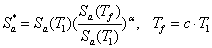,                        (1)

where a and c are two parameters to be calibrated.

Note that the dynamic behavior of buildings upon entering into the nonlinear stage is not fully understood, particularly at the early stage of structural design because of the difficulties in accurately predicting the nonlinear behavior of the building. Consequently, IMs that consider period elongation are limited in scope and practical application.

With respect to the higher vibration modes, the IM proposed by Vamvatsikos and Cornell (2005) also considers the participation of higher-order vibration modes. In the expression, the values of ta and tb represent the higher modal periods of concern.

Similarly, Luco and Cornell (2007) proposed an IM called IM1E&2E based on the first two vibration modes and the square-root-of-sum-of-squares (SRSS) rule of modal combination. This IM considers the effect of the contribution of higher modes caused by the near-fault pulse, as expressed in Eqn 2.

where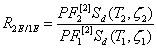and Sd(T1,z1) and Sd(T2,z2) are the elastic spectral displacements, respectively, at damping ratios z1 and z2 with corresponding periods T1 and T2. Eqn 2 also defines the jth ¨C mode participation factor for the story drift ratio of the ith story, qi, as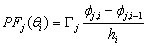. Note that PF 1 denotes the first mode participation factor for the maximum peak inter-story drift angle, qmax, obtained using the SRSS of the first two modes. Similarly, PF 1 represents the first-mode participation factor for qmax when only the first mode is considered. The coefficient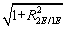reflects the effect of the second mode on qmax and the relevant spectral shape. The coefficient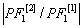mainly indicates the influence of the first-mode and first-two-modes estimations on qmax, which may occur on different stories. The earlier proposed IM1E&2E has also been improved by the same researchers (Luco and Cornell 2007), where the inelastic spectral displacement SI d(T1,z1,dy) was adopted as a replacement for the elastic spectral displacement Sd(T1,z1). The new IM is called IM1I&2E. Despite the advancement of IM1E&2E and IM1I&2E, their complex expressions render the various parameters difficult to be determined, especially at the early design stage, thus limiting practical application of these IMs.

In addition to the aforementioned scalar IMs, some vector-valued IMs have also been proposed in recent years. For example, Baker and Cornell (2005) proposed a two-parameter vector-valued IM called <Sa(T1), e>. The definition of parameter e is originated from seismology engineering. This parameter reflects not only the distinction of the spectral shapes but also, to some extent, the effect of higher-mode contribution and period elongation. However, due to the absence of the basic data required to obtain e in many countries, more work must be performed before the vector-valued IM <Sa(T1), e> can be applied worldwide.

Ye et al. (2013) compared the applicability of 18 commonly used IMs in an elasto-plastic analysis of different buildings. The findings indicated that Sa(T1) has the best correlation with the structural demand measures (DMs) for short- and moderate-period structures. In addition, the correlation between PGV and the DMs is also acceptable. Furthermore, PGV performs better as an IM when the fundamental period of the structure increases. Based on their study, Sa(T1) and PGV have been recommended for use as the IMs in actual structural design.

Existing research on IMs as outlined above has mainly targeted structures whose fundamental periods are shorter than 6 s and mostly in the range of 0 to 4 s. It is evident that the rationality of the existing IMs requires further validation for super high-rise buildings with fundamental periods as long as approximately 10 s. This validation is the focus of the present study, with an emphasis on the actual requirements of structural design. A total of six different IMs (PGA, PGV, PGD, Sa(T1), Sd(T1), and IM1E&2E) are evaluated and their rationalities are discussed. PGV and Sa(T1) were selected based on the recommendations of Ye et al. (2013). To consider the contribution of the higher-order vibration modes of super high-rise buildings, IM1E&2E was also selected. In addition, PGA, PGD and Sd(T1) were considered because they are widely used in actual design. The correlation between the IMs and DMs with different vibration periods is analyzed, and rational IMs for super high-rise building design are recommended.

3. ANALYTICAL MODEL AND GROUND MOTION SELECTION

3.1 Simplified analytical model

This study mainly focuses on super high-rise buildings with very long fundamental vibration periods. In general, the commonly used lateral seismic-resistant super high-rise systems include framed tube, bundled tube, tube-in-tube, diagonalized (trussed tubes, diagrids or braced frames), core and outrigger (central lateral system linking to the perimeter system through outriggers) and hybrid systems (Lu et al. 2011). The deformation modes of these structural systems are typically a combination of flexure and shear, which differs greatly from the traditional frame or shear wall buildings. This implies that the traditional multistoried shear model and cantilever flexural beam model are not suitable for super high-rise buildings. Consequently, the dynamic properties and seismic responses of super high-rise buildings are approximated herein by using an equivalent continuum model consisting of a shear and a flexural cantilever beam, which is proposed by Miranda and Taghavi (2005) (Figure 1). The shear and flexural cantilever beams are connected by an infinite number of rigid links to achieve horizontal deformation compatibility at the same height. The response of this model subjected to ground acceleration can be expressed in the following partial differential equation:,          (5)

where u(x,t) is the horizontal displacement at non-dimensional height x at time t; m(x) is the mass per unit length of the model; x varies between 0 at the base of the building and 1 at the roof level; ug(t) represents ground displacement at time t; H denotes the total height of the model; and EI(x) and GA(x) are, respectively, the flexural stiffness of the flexural beam and the shear stiffness of the shear beam at non-dimensional height x. Miranda and Taghavi (2005) assumed that the variations in the shear and flexural stiffness along the height are identical, i.e., S(x) or

 EI(x)=EI0S(x) (6) GA(x)=GA0S(x) (7)

in which, S(x) is the dimensionless function that defines the variation of stiffness along the height of the simplified model, and EI0 and GA0 are, respectively, the flexural and shear stiffness at the base of the model. The dimensionless parameter a0=H(GA0/EI0)1/2 controls the proportions of the flexural and shear deformations in the simplified model and thus the lateral deformation shape of the building. Theoretically, when a0=0, the model degenerates into a pure bending model, and when a0=¡Þ, it degenerates into a pure shear model. Miranda and Taghavi (2005) indicated that a0 is normally between 0 and 1.5 for shear wall buildings; between 1.5 to 5 for buildings with dual structural systems consisting of a combination of moment-resisting frames and shear walls; and between 5 and 20 for moment-resisting frame buildings. In addition, when a0¡30, the deformation mode is dominated by shear deformations. A number of different buildings were analyzed by Miranda and Taghavi (2005) to verify the appropriateness of the model in simulating the elastic vibration of different buildings.

To ensure the appropriateness of the simplified model, the 632 m super high-rise building Shanghai Tower (see Figure 2) is selected herein to calibrate the key parameters used in the model. The Shanghai Tower has a hybrid lateral seismic-resistant system called the ¡°mega-column/core-tube/outrigger-truss¡±. According to architecture and functional requirements, eight reinforced stories were built for every 13 to 16 stories, which divide the entire structure into eight zones. The plane layouts of these eight stories are also briefly illustrated in Figure 2. The fundamental period of the Shanghai Tower is nearly 9.83 s (Lu et al. 2011), which is far beyond the range of 6 s specified in the design response spectrum of the Chinese Code for the Seismic Design of Buildings (MOC 2010).

The parameters of the simplified model are calibrated such that the fundamental dynamic properties of the simplified model approach those of the Shanghai Tower in the XZ plane. The total gravitational load of the simplified model, which is determined from a combination of 1.0 D + 0.5 L, is identical to that of the Shanghai Tower, in which D is the dead loads, including the effects of self weight and permanently attached equipment and fixtures; L is the live loads. Note that the dead and live loads of the Shanghai Tower have little variation along its height; the simplified model thus has a constant mass distribution along the height. According to the stiffness distribution of the Shanghai Tower, a quadratic curve, i.e., S(x)=1-(1-0.55)x2, is adopted to model the structural stiffness along the height (Eqns 6 and 7). The flexural stiffness at the base, EI0, and the dimensionless flexural and shear ratio, a0, are calibrated according to the dynamic properties of the Shanghai Tower.

The values of the main parameters used in the simplified model are summarized in Table 1. The first five free translational vibration periods and the relative deviation D between the simplified model and the fine FE model of Shanghai Tower are compared in Table 2. The relative deviation D, defined by Eqn 8, is adopted to describe the distinction between the two models. In the equation, Tsimplify and Tfine represent, respectively, the free translational vibration periods of the simplified model and the fine FE model of Shanghai Tower.

 D = (Tsimplify - Tfine) / Tfine (8)

It is evident that some discrepancies are present in the comparison. Note that a substantial reduction in computational costs is achieved by employing the simplified model compared with a very fine FE model. Note also that the focus of this study is to offer a general rule for super high-rise buildings instead of investigating the characteristics of a particular structure. Therefore, the resulting discrepancies are considered acceptable.

 3.2 Ground motion selection Due to significant randomness in the ground motion records, the structural responses may vary significantly in a time-history analysis. To minimize such a variation while maintaining inherent randomness of the ground motions, 22 pairs of far-field ground motion records recommended by the FEMA P695 (FEMA 2009) are adopted herein as the basis ground motion record set. Note that two horizontal components of each ground motion are considered, thereby resulting in 44 natural records. Their corresponding elastic acceleration spectrums at a 5% damping ratio are presented in Figure 3. This ground motion record set not only maintains the randomness of the ground motions themselves but also leads to a certain degree of consistency in the structural responses. 4. EVALUATION OF THE INTENSITY MEASURES 4.1 Evaluation method The existing literature (Baker and Cornell 2005, 2008; Padgett et al. 2008) indicates that the relationship between the structural seismic response demand measures (DMs) and the ground motion intensity measures (IMs) can be estimated using the power-function model presented in Eqn 9: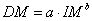,                             (9) in which a and b are the regression coefficients. The equation can be rearranged in a linear regression form of logarithms of DM and IM; i.e.,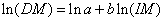(10) Eqn 10 clearly satisfies the classical linear regression model. The correlation coefficient r  between ln(DM) and ln(IM) and the corresponding dispersion b can then be obtained by regression analysis of n discrete data points (DMi, IMi). This analysis can be performed using the least square method in time-history analysis. The value of dispersion b is calculated from Eqn 11 below:(11) The correlation coefficient r  ranges between -1 and 1. The closer to 1 |r| is, the better the correlation between the calculated DMs and IMs. In general, |r|¡0.8 implies a good correlation. In addition, the smaller the dispersion b, the better the IMs are. Note that all modern-day super high-rise buildings are rigorously designed with sufficient safety margins to meet the seismic requirement under the Maximum Considered Earthquake (MCE) specified in the design codes. Thus, the major lateral force resisting components remain elastic or slight yielding, instead of exhibiting obvious nonlinear behavior, when subjected to MCE. Therefore, elastic time history analysis is considered appropriate in evaluating the rationality of the existing IMs for super high-rise buildings. The specific research procedures are as follows: (1) For a simplified model with a given fundamental period and corresponding parameters, calculate the maximum structural response DMi subjected to the ith ground motion record using the time-history analysis method; (2) Calculate the IMi of the corresponding ith ground motion record; (3) Calculate n ground motion records and obtain n discrete data points (IMi£¬DMi) and plot them in the ln(IM)-ln(DM) coordinate system. The correlation coefficient r between ln(IM) and ln(DM) for the given fundamental period and the corresponding dispersion b are obtained by linear regression. The schematic diagram of correlation between the IM and DM is shown in Figure 4; (4) Adjust the model parameters (EI0 or r(x)) to obtain a series of structures with different fundamental periods. Next, repeat steps 1 to 3 to calculate the variations in the correlation coefficient between DMi and IMi and in the corresponding dispersion for different structural fundamental periods. Note that although only EI0 or r(x) is modified in this step, the influence of adjusting other parameters will be discussed in later sections of this work. While many DMs are available, such as the maximum top displacement (dmax), maximum acceleration (amax), maximum story drift ratio (qmax), maximum base shear force (Fmax), and total input energy (Einput), the most commonly used DMs in structural seismic design and seismic response analysis are qmax, dmax and amax. Therefore, this paper aims to discuss the variations in the correlation coefficients between these DMi (qmax, dmax, amax) and IMi and their corresponding dispersion with different structural fundamental periods T1. 4.2 Result Analysis Based on the simplified model with a0=4 proposed in the above section in Table 1, five different simplified models with the fundamental periods of 1, 3, 6, 9 and 10 s respectively are established by adjusting the values of EI0 and r(x). In these models, classical Rayleigh damping is adopted with a value of 5%. Figures 5 to 10 demonstrate the variations in correlation between the DMs of interest (qmax, dmax, amax) and the IMs (PGA, PGV, PGD, Sa(T1), Sd(T1), and IM1E&2E) and their corresponding dispersion for different fundamental periods. Figure 5 demonstrates that the correlation between PGA, Sa(T1), Sd(T1), and IM1E&2E and qmax decrease gradually with an increase at the fundamental period, particularly for PGA. When the fundamental period approaches 10 s, the correlation coefficient of PGA is less than 0.4, which indicates that PGA has little correlation with qmax for the structures with super long periods. In addition, the correlation coefficient for IM1E&2E is approximately 0.7, which indicates that IM1E&2E has some correlation with qmax; however, it does not accurately represent the characteristic of the seismic response of super high-rise buildings because only the first two modes are considered. The correlation between PGV and PGD and qmax increase gradually with increasing fundamental period, and after 6 s, the correlation between PGV and PGD and qmax decreases slightly. In addition, for a fundamental period of 9 s, the correlation coefficient r of PGD increases to 0.6, while the correlation coefficient r of PGV approaches 0.84. Therefore, PGV has the best correlation with qmax, and PGA has the least correlation with qmax at a period of 10 s. Similarly, Figure 6 indicates that in the range of 10 s, the dispersion of PGV is the smallest, while the dispersion of PGA is the largest. These results indicate that for super high-rise buildings, PGV has the best correlation with qmax and the minimum dispersion. The existing literature also suggests that qmax has a significant correlation with structural damage (Bozorgnia and Bertero 2001; Miranda and Akkar 2006). This further confirmed that PGV can be suggested as an IM for structural seismic design and collapse analysis for super high-rise buildings. Figure 7 indicates that Sa(T1), Sd(T1) and IM1E&2E have the greatest correlation with dmax, and the correlation coefficients always remain at approximately 0.94, even when the fundamental period increases from 1 to 10 s. The correlation between PGD and dmax increases gradually from less than 0.25 (at a period of 1 s) to 0.94 (at a period of 10 s). The correlation between PGV and dmax decreases gradually, and the correlation coefficient r is approximately 0.48 at a period of 10 s. Finally, the correlation between PGA and dmax decreases rapidly with increasing period and even becomes negative when the fundamental period is equal to 10 s. Figure 8 also indicates similar results, i.e., Sa(T1), Sd(T1) and IM1E&2E have the minimum dispersion among these IMs. Figures 9 and 10 demonstrate that PGA has the greatest correlation with the structural maximum acceleration (amax) and the smallest dispersion b; and the value of the correlation coefficient r remains at approximately 0.85 when the fundamental period increases from 1 to 9 s. PGV also shows some correlation with amax,, and the correlation coefficient r remains near 0.6 and increases slightly with an increasing period. In contrast, Sa(T1), Sd(T1) and IM1E&2E display poor correlations with amax with large dispersions b. The correlation coefficients decrease rapidly with an increase in the fundamental period. When the fundamental period becomes very long, the correlation coefficients become negative. This implies that the two variations have very little correlation, in other words, the more the IMs (Sa(T1), Sd(T1) and IM1E&2E) are, the smaller the amax is. In addition, PGD also shows little or no correlation with amax and the correlation coefficients is close to 0 in the period range concerned. In performance-based design, acceleration is usually associated with comfort and property loss; therefore, PGA can be selected as the IM for structural acceleration prediction. 4.3 Influence of a0 To make the aforementioned results universal and representative, the influence of a0 on the simplified model is discussed. Miranda and Taghavi (2005) reported that when a0=0, the simplified model degenerates into a pure bending model, whereas when a0=¡Þ, this model can be approximately considered as a pure shear model. Although pure bending or pure shear behavior have little possibility of occurrence in super high-rise buildings with a vibration period as long as 9 s, for investigation purpose, a series of simplified models with a0=0 and 30 and T1=1, 3, 6, 9, and 10 s (totally 10 simplified models) are created to examine the relationship between a0 qmax and each IM when a0=0 are illustrated in Figures 11 and 12, respectively. In addition, similar variations in the correlation and dispersion when a0=30 are illustrated in Figures 13 and 14, respectively. For different values of a0, Figures 15 and 16 demonstrate, respectively, the correlation coefficient r and the corresponding dispersion b between PGV and qmax. When a0=0, the simplified model represents a pure bending structure, whose higher-order period decreases rapidly. The second and third vibration periods (i.e., T2 and T3) are approximately 1/6 and 1/16 of the fundamental period T1, respectively. For the flexural/shear structures with a0=4, on the other hand, T2 and T3 are approximately 1/3 and 1/6 of T1, respectively. It is evident that the influence of higher-order modes is smaller for pure bending structures. However, IM1E&2E, which considers the first two vibration modes, has a significant correlation with qmax. Figures 11 and 12 indicate that the correlation coefficients decrease slightly with increasing T1, and the values of the correlation coefficients remain above 0.9 for different fundamental periods. The dispersion of IM1E&2E is also the smallest. The correlation coefficients between PGV and qmax at different fundamental periods when a0=0 are always above 0.7. The correlation between Sa(T1) (or Sd(T1)) and qmax when a0=0 decreases gradually when T1 increases. When T1 is shorter than 6 s, the correlation between Sa(T1) (or Sd(T1)) and qmax is slightly greater than that of PGV; however, when T1 is longer than 6 s, the correlations are slightly worse than those of PGV. The correlation between PGD and qmax increases rapidly with increasing T1, and when T1 is longer than 6 s, the correlation decreases slightly, which is similar to the variation in PGV. PGA exhibits the least correlation with qmax, and the correlation coefficients decrease rapidly with increasing T1. When T1 approaches 10 s, the correlation coefficient increases slightly, but the value is still very low at approximately 0.27. When a0= 30, the variations in the correlation coefficient r and the corresponding dispersion b  between qmax and each IM at different fundamental periods approximate the values when a0=4. That is, by increasing the structural fundamental period, the correlations between the IMs (PGA, Sa(T1), Sd(T1), and IM1E&2E) and qmax decrease gradually, while the correlations between PGV (or PGD) and qmax increase steadily. For a super long-period structure with a fundamental period longer than 9 s, PGV exhibits the best correlation with qmax and minimum dispersion. In contrast, PGA exhibits the least correlation with qmax and maximum dispersion. Furthermore, Figures 15 and 16 demonstrate that for a pure bending structure (a0=0), the correlation coefficients between PGV and qmax at different fundamental periods are smaller than the values of a flexural-shear deformation combined structure (a0=4) or a shear deformation dominant structure (a0=30). For the bending-shear deformation combined structure (a0=4), the correlation coefficients are slightly smaller than the values for the shear deformation dominant structure (a0=30) if the fundamental period T1 is shorter than 5 s. However, when T1 is longer than 5 s, the correlation coefficients are slightly larger than the values for the shear deformation dominant structure (a0=30). Moreover, when T1 approaches 10 s, these values are very similar. Overall, for different values of a0, PGV always shows a good correlation with qmax. Considering that in practical application, most super high-rise buildings are flexural-shear deformation combined systems, it is still feasible to select PGV as the IM for the structural seismic design and collapse analysis of super high-rise buildings. Similarly, for different values of a0, Figures 17 and 18 demonstrate, respectively, the variations of the correlation coefficient r and the corresponding dispersion b between Sa(T1) and dmax; and such variations between PGA and amax are presented in Figures 19 and 20, respectively. Figure 17 illustrates that a0 has little influence on r between Sa(T1) and dmax, where r is constantly above 0.95 for different values of a0 in the period range concerned. The corresponding b remains below 0.2 (see Figure 18). Figure 19 illustrates that best correlations are achieved between PGA and amax for shear deformation dominant structures (a0=30), and this is followed by flexural-shear deformation combined structures (a0=4). Whereas smallest correlation is found for pure bending structures (a0=0). Such findings suggest that the larger the value of a0 is, the better the correlation is between PGA and amax. It is necessary to note that the smallest correlation coefficient for pure bending structures (a0=0) is still larger than 0.78. This implies that PGA correlates very well with amax when a0 varies between 0 and 30; and the influence of a0 on the correlation coefficients is insignificant. In general, a0 has little influence on the correlation between IMs and DMs. Although the discussions given in Section 4.2 are based on the analysis of the Shanghai Tower, the findings remains universal and are applicable to other similar super high-rise buildings. In summary, for different values of a0, PGV, Sa(T1) and PGA always demonstrate good correlations with qmax, dmax and amax, respectively.
 5. VERIFICATION THROUGH COLLAPSE ANALYSIS OF THE SHANGHAI TOWER It should be noted that the maximum story drift ratio qmax is always the controlling factor for seismic safety measure of building structures. Due to a very good correlation between PGV and qmax, as indicated in the above analysis, PGV could be selected as an IM for seismic design and response prediction of super high-rise buildings. This conclusion is reached based on the simplified model illustrated in Figure 1 with elastic dynamic analysis; however, a super high-rise building may enter the nonlinear stage or even collapse under extreme earthquake conditions. Hence, the rationality of this conclusion must be further validated when the super high-rise building enters the nonlinear region. For this reason, the following section presents a verification of the above conclusion using an incremental dynamic collapse analysis of the Shanghai Tower presented in Figure 2. Lu et al. (2011, 2013a, 2013b) proposed a nonlinear finite element model based on the general purpose finite element code of MSC.Marc to simulate the collapse of the high-rise and super high-rise buildings, including the Shanghai Tower. In the FE model of Shanghai Tower, the fiber model is adopted to simulate the columns and beams in the external frame and the components of the outriggers. The multi-layer shell model is used to simulate the shear walls, the coupling beams and the mega columns; and elemental deactivation technology is adopted to simulate the failure of the components upon reaching their ultimate deformation stage. The complete FE model of the Shanghai Tower is illustrated in Figure 2. Further details of the structural dimensions, the material constitutive relationships and the collapse simulation procedures can be found in Lu et al. (2011). Incremental dynamic analysis (IDA) is a powerful method in earthquake engineering for performing a comprehensive assessment of the structural behavior under seismic loads. During IDA, several ground motions are considered with increased levels of seismic intensity thereby making the structure undergo an entire range of behavior, from elastic to inelastic and finally to collapse. In this paper, the larger components of each of the 22 pairs of far-field ground motion records suggested by FEMA P695 (FEMA 2009) are adopted as the ground motion set. In addition, data from the El-Centro EW 1940 ground motion record and the Shanghai artificial ground motion record are added into this ground motion set. This makes a total of 23 natural records and 1 artificial record. During the analysis, these ground motion records are input into the fine FE model along the X direction and their intensities are gradually increased up to collapse of the building. One of the typical potential collapse modes is shown in Figure 21. The values of different IMs (PGA, PGV, PGD, Sa(T1), Sd(T1), and IM1E&2E) that cause the building to collapse are presented in Table 3. Since this building is very important, its safety margin against earthquake-induced collapse is very large. The coefficient of variation (COV) of each IM is presented in Figure 22. It can be seen that when the building begins to collapse under the 24 ground motion records, the COVs of Sd(T1) and Sa(T1) are maximum, reaching nearly 0.729 and 0.690. This is followed by the PGA, PGD and IM1E&2E. In addition, PGV has the minimum COV, i.e. 0.182, which is approximately 2/5 of the COV of PGA and 1/4 of the COV of Sa(T1). Even when the super high-rise building enters the nonlinear stage, PGV still shows a very good correlation with the structural response and collapse possibility. PGV can therefore be selected as the IM for seismic response prediction and collapse analysis.

6. CONCLUSIONS

This paper briefly reviewed some existing IMs and constructed a simplified flexural-shear beam-coupling model based on an actual super high-rise building, the Shanghai Tower. The correlations between the fundamental DMs and some existing IMs of this super high-rise building are analyzed, and the appropriate scopes for different IMs are also discussed. Consequently, the following preliminary conclusions are obtained:

(1) With increasing structural fundamental period, the correlations between PGA, Sa(T1), Sd(T1) and the maximum story drift ratio (qmax) decrease rapidly, and their corresponding dispersions increase gradually. Hence, PGA, Sa(T1) and Sd(T1) are not suitable IMs for super high-rise buildings for seismic design and time history analysis.

(2) In the range of super long periods, the correlation coefficient between PGV and qmax is more than 0.8 with a smallest dispersion. These results indicate that PGV has a significant correlation with qmax. Therefore, PGV can be adopted as the IM for super high-rise buildings with super long periods.

(3) Over the range of short to super long periods, Sa(T1) and Sd(T1) always exhibit significant correlations with the structural maximum top displacement (dmax); therefore, Sa(T1) and Sd(T1) can be selected as the IMs for structural global displacement prediction.

(4) PGA exhibits a significant correlation with the structural acceleration response; therefore, PGA can be selected as the IM for structural acceleration prediction and comfort design.

(5) From the collapse analysis of the Shanghai Tower, it can be concluded that under strong or extreme earthquakes, even when the super high-rise building enters the nonlinear region, PGV can still be selected as the IM for seismic response prediction and collapse analysis.

It is worth mentioning that the above findings are reached based on the investigation of a single building ¨C the Shanghai Tower. To ascertain these research findings to be used for seismic design guidelines of super high-rise buildings, more case studies on other tall buildings are desirable in further work.

ACKNOWLEDGMENT

The authors are grateful for the financial support received from the National Nature Science Foundation of China (No. 51222804, 51261120377, 51178249), the Tsinghua University Initiative Scientific Research Program (No. 2012THZ02-2, 2011THZ03) and the Fok Ying Dong Education Foundation (No. 131071).

References

Baker, J.W. and Cornell, C.A. (2005). ¡°A vectored-valued ground motion intensity measure consisting of spectral acceleration and epsilon¡±, Earthquake Engineering and Structural Dynamics, Vol. 34, No. 10, pp. 1193-1217.

Baker, J.W. and Cornell, C.A. (2008). ¡°Vector-valued intensity measures for pulse-like near-fault ground motions¡±, Engineering Structures, Vol. 30, No. 4, pp. 1048-1057.

Bozorgnia, Y. and Bertero, V.V. (2001). ¡°Improved shaking and damage parameters for post-earthquake applications¡±, Proc., SMIP01 Seminar on Utilization of Strong-Motion Data, California Division of Mines and Geology, Los Angeles, pp. 1-22.

Cordova, P.P., Deierlein, G.G., Mehanny, S.S.F. and Cornell, C.A. (2001). ¡°Development of a two-parameter seismic intensity measure and probabilistic assessment procedure¡±, The Second U.S.-Japan Workshop on Performance-based Earthquake Engineering Methodology for Reinforced Concrete Building Structures, Sapporo, Japan, pp. 187-206.

FEMA (2009). FEMA P695: Quantification of building seismic performance factors, Applied Technology Council, Redwood City, C.A.

Lu, X.Z., Lu, X., Guan, H., Zhang, W.K. and Ye, L.P. (2013). ¡°Earthquake-induced collapse simulation of a super-tall mega-braced frame-core tube building¡±, Journal of Constructional Steel Research, Vol. 82, pp. 59-71.

Lucchini, A., Mollaioli, F. and Monti, G. (2011). ¡±, Bulletin of Earthquake Engineering, Vol. 9, No.5, pp. 1499-1518.

Luco, N. and Cornell, C.A. (2007). ¡°Structure-specific scalar intensity measures for near-source and ordinary earthquake ground motions¡±, Earthquake Spectra, Vol. 23, No. 2, pp. 357-391.

MOC (2010). Code for seismic design of buildings GB50011-2010, Ministry of Construction of the People¡¯s Republic of China, Beijing, China.

Miranda, E. and Akkar, S.D. (2006). ¡°Generalized interstory drift spectrum¡±, Journal of Structural Engineering, ASCE, Vol. 132, No. 6, pp. 840-852.

Miranda, E. and Taghavi, S. (2005). ¡°Approximate floor acceleration demands in multistory buildings I: Formulation¡±, Journal of Structural Engineering, ASCE, Vol. 131, No. 2, pp. 203-211.

Padgett, J.E., Nielson, B.G. and DesRoches, R. (2008). ¡°Selection of optimal intensity measures in probabilistic seismic demand models of highway bridge portfolios¡±, Earthquake Engineering and Structural Dynamics, Vol. 37, No. 5, pp. 711-725.

Shome, N., Cornell, C.A., Bazzurro, P. and Carballo, J.E. (1998). ¡°Earthquakes, records and nonlinear responses¡±, Earthquake Spectra, Vol. 14, No. 3, pp. 469-500.

The Building Centre of Japan. (2000). ¡°The building standard law of Japan¡±, Japan.

Tothong, P. and Luco, N. (2007). ¡°Probabilistic seismic demand analysis using advanced ground motion intensity measures¡±, Earthquake Engineering and Structural Dynamics, Vol. 36, No. 13, pp. 1837-1860.

Vamvatsikos, D. and Cornell, C.A. (2005). ¡°Developing efficient scalar and vector intensity measures for IDA capacity estimation by incorporating elastic spectral shape information¡±, Earthquake Engineering and Structural Dynamics, Vol. 34, No.13, pp. 1573-1600.

Yang, X.Q., Fu ,X.Y. and Huang, Y.J. (2011). ¡°Dynamic elasto-plastic analysis of the Shenzhen Ping¡¯an Financial Center Tower¡± (in Chinese). Journal of Building Structures, Vol. 32, No.7, pp.40-49.

Ye, L.P., Ma, Q.L., Miao, Z.W., Guan, H. and Zhuge, Y. (2013). ¡°Numerical and comparative study of earthquake intensity indices in seismic analysis¡±, The Structural Design of Tall and Special Buildings, Vol. 22, No. 4, pp.362-381.

List of Tables

List of figures

Figure 5.                   The variations of correlation between qmax and IMs at different fundamental periods

Figure 6.                   The variations of dispersion between qmax and IMs at different fundamental periods

Figure 11.               The variations of correlation between qmax and IMs at different fundamental periods when a0=0

Figure 12.               The variations of dispersion between qmax and IMs at different fundamental periods when a0=0

Figure 13.               The variations of correlation between qmax and IMs at different fundamental periods when a0=30

Figure 14.               The variations of dispersion between qmax and IMs at different fundamental periods when a0=30

Figure 15.               The variation of correlation coefficients between PGV and qmax with different value of a0

Figure 16.               The variation of dispersion between PGV and qmax with different value of a0

Figure 18.               The variation of dispersion between Sa(T1) and dmax with different value of a0

Figure 19.               The variation of correlation coefficients between PGA and amax with different value of a0

Figure 20.               The variation of dispersion between PGA and amax with different value of a0

Figure 21.               The potential collapse mode of the Shanghai Tower (The failed elements are removed from the model)

Figure 22.               The distribution of COV for different IMs at the stage of collapse

Appendix. Notation

The following symbols are used in this paper:

IM                 ground motion intensity measure

DM              seismic response demand measure

PGA              the peak ground motion acceleration

PGV             the peak ground motion velocity

PGD             the peak ground motion displacement

Sa(T1)            the spectral acceleration at fundamental period

Sd(T1)            the spectral displacement at fundamental period

dmax              the maximum top displacement

amax                    the maximum acceleration

qmax             the maximum story drift ratio

IDA               incremental dynamic analyses

THA              time-history analysis

a0                   the dimensionless parameter controls the proportions of the flexural and shears deformations

r                     the correlation coefficient  between DM and IM

b                     the dispersion coefficient  between DM and IM

COV             the coefficient of variation

Table 1.  Main parameters used in the simplified model

 Gravity load / ton EI0 / N•m2 a0 Damping ratio z 6.88¡°108 2.4¡°1014 4.0 5%

Table 2.  Comparison of the first five periods between the simplified model and the fine FE model

 T1 T2 T3 T4 T5 The fine FE model (s) 9.83 3.57 1.67 1.02 0.72 The simplified model (s) 9.85 3.25 1.71 1.05 0.70 Relative deviation D -0.23% -8.9% 2.87% 3.18% -2.60%

Table 3.  The values of different IMs at the critical collapse status of the Shanghai Tower

 PGA (g) PGV (cm/s) PGD (cm) Sa(T1) (g) Sd(T1) (cm) IM1E&2E (g) CAPEMEND_RIO270 2.2 249.92 122.34 0.0766 179.48 0.6024 CHICHI_CHY101-E 1.0 200.03 128.25 0.0830 193.56 1.3065 CHICHI_TCU045-E 2.8 216.48 298.92 0.2494 591.10 1.9549 DUZCE_BOL000 3.7 287.04 117.25 0.0988 226.69 0.9899 FRIULI_A-TMZ000 4.9 307.11 57.45 0.0327 66.57 0.4374 HECTOR_HEC000 2.2 236.55 186.39 0.1568 369.28 1.2333 IMPVALL_H-DLT262 1.9 207.61 95.63 0.1243 293.62 1.6218 IMPVALL_H-E11140 2.6 245.97 113.06 0.0762 179.00 1.2303 KOBE_NIS000 3.8 278.04 71.02 0.0473 104.36 0.5727 KOBE_SHI000 1.6 248.89 56.17 0.0315 67.93 0.6726 KOCAELI_ARC000 2.8 226.24 174.49 0.2093 495.39 1.6524 KOCAELI_DZC180 0.8 150.69 112.93 0.1070 251.70 0.8599 LANDERS_CLW-LN 2.4 217.43 116.41 0.1224 287.30 0.9601 LANDERS_YER270 0.7 146.99 125.11 0.1153 272.45 0.9064 LOMAP_CAP000 3.4 225.12 58.94 0.0362 70.57 0.4070 LOMAP_G03000 4.6 295.63 68.18 0.0518 110.49 0.6404 MANJIL_ABBAR--L 2.6 218.35 76.15 0.0563 123.70 0.5656 NORTHR_LOS000 2.0 209.65 56.91 0.0330 63.70 0.5564 NORTHR_MUL009 2.0 283.33 63.17 0.0323 65.47 0.5090 SFERN_PEL090 2.2 198.35 130.36 0.0808 187.77 1.1285 SUPERST_B-ICC000 2.0 258.85 98.17 0.1247 293.54 0.9823 SUPERST_B-POE270 2.8 224.43 55.26 0.0286 57.11 0.7038 El-Centro 1.8 201.98 48.07 0.0359 79.42 0.6422 Shanghai 1.4 182.75 92.94 0.0311 63.61 0.4805 COVs 0.443 0.182 0.530 0.690 0.729 0.468Figure 1.  The simplified model consisting a flexural and a shear beam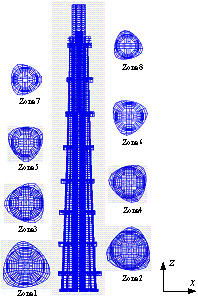Figure 2.  The FE model of Shanghai Tower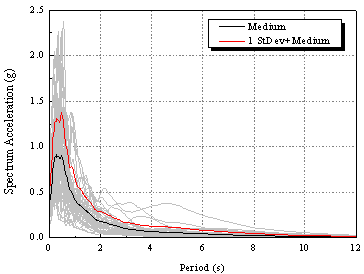Figure 3.  The elastic acceleration spectrum of the 44 far-field ground motions at a 5% damping ratio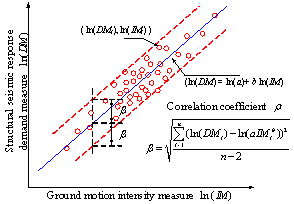Figure 4.  The schematic diagram of correlation between the IM and DM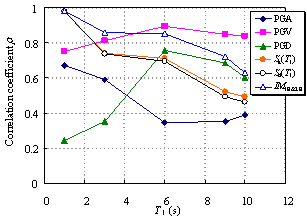Figure 5.  The variations of correlation between qmax and IMs at different fundamental periods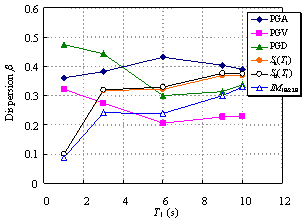Figure 6.  The variations of dispersion between qmax and IMs at different fundamental periods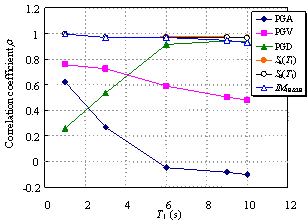Figure 7.  The variations of correlation between dmax and IMs at different fundamental periods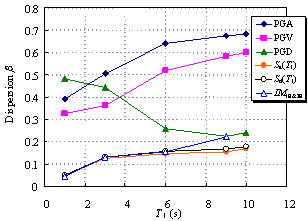Figure 8.  The variations of dispersion between dmax and IMs at different fundamental periodsFigure 9.  The variations of correlation between amax and IMs at different fundamental periods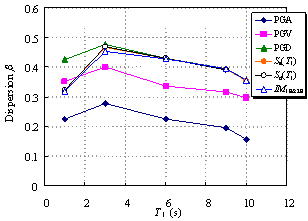Figure 10.  The variations of dispersion between amax and IMs at different fundamental periods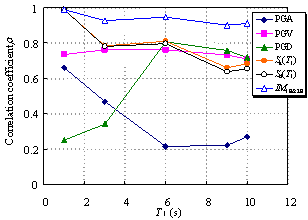Figure 11.  The variations of correlation between qmax and IMs at different fundamental periods when a0=0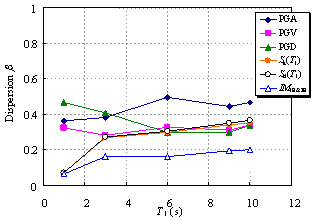Figure 12.  The variations of dispersion between qmax and IMs at different fundamental periods when a0=0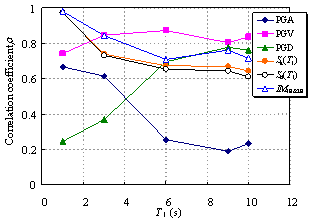Figure 13.  The variations of correlation between qmax and IMs at different fundamental periods when a0=30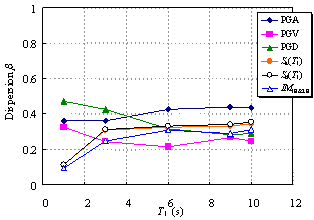Figure 14.  The variations of dispersion between qmax and IMs at different fundamental periods when a0=30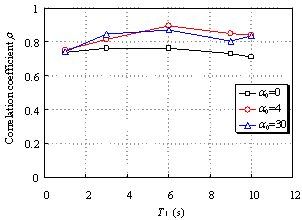Figure 15.  The variation of correlation coefficients between PGV and qmax with different value of a0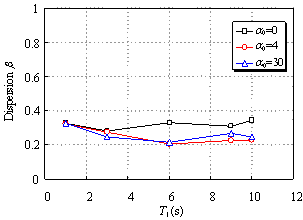Figure 16.  The variation of dispersion between PGV and qmax with different value of a0Figure 17.  The variation of correlation coefficients between Sa(T1) and dmax with different value of a0Figure 18.  The variation of dispersion between Sa(T1) and dmax with different value of a0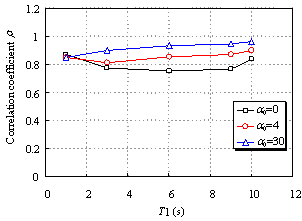Figure 19.  The variation of correlation coefficients between PGA and amax with different value of a0Figure 20.  The variation of dispersion between PGA and amax with different value of a0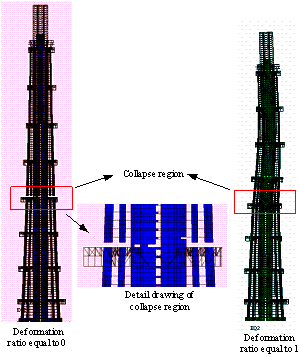Figure 21.  The potential collapse mode of Shanghai Tower (The failed elements are removed from the model)Figure 22.  The distribution of COV for different IMs at the stage of collapse

[*] Corresponding author. E-mail address: luxz@tsinghua.edu.cn. Tel: +86-10-62795364; fax: +86-10-62795364